Courses

# NCERT Solutions(Part- 4)- Linear Equations in One Variable Class 8 Notes | EduRev

## Class 8 Mathematics by VP Classes

Created by: Vp Classes

## Class 8 : NCERT Solutions(Part- 4)- Linear Equations in One Variable Class 8 Notes | EduRev

The document NCERT Solutions(Part- 4)- Linear Equations in One Variable Class 8 Notes | EduRev is a part of the Class 8 Course Class 8 Mathematics by VP Classes.
All you need of Class 8 at this link: Class 8

EXERCISE 2.4

Ques 1: Amina thinks of a number and subtracts 5/2 from it. She multiplies the results by 8. The result now obtained is 3 times the same number she thought of. What is the number?
Ans: Let the number be ‘x’.

∴ Subtracting 5/2 , we get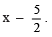According to the condition, we have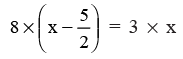or

8x – 20 = 3x

Transposing –20 to RHS, we have

Again transposing 3x to LHS, we have

8x – 3x = 20
or

5x = 20

or

x = 20/5 = 4

Thus, required number = 4

Ques 2: A positive number is 5 times another number. If 21 is added to both the numbers, then one of the new numbers becomes twice the other new number. What are the numbers?
Ans: Let positive number = 5x

∴ The other number = x

On adding 21 to both number:
We get (5x + 21) and (x + 21)
According to the condition, we have

2 * (x + 21) = 5x + 21

or

2x + 42 = 5x + 21

Transposing 42 to RHS, we have

2x = 5x + 21 – 42

Transposing 5x to LHS, we have 2

2x – 5x = –21

or

–3x = –21

Dividing both sides by (–3), we have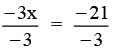or

x = 7
and  5x = 5 * 7 = 35

Thus, the required numbers are 7 and 35.

Ques 3: Sum of the digits of a two-digit number is 9. When we interchange the digits, it is found that the resulting new number is greater than the original number by 27. What is the two-digit number?
Ans: Let the unit’s digit = x
∴  The ten’s digit = (9 – x)
∴  The original number = 10(9 – x) + x
= 90 – 10x + x
= 90 – 9x

On interchanging the digits, the new number = 10x + (9 – x)
= 10x + 9 – x
= 9x + 9

According to the condition, we have have

[New number] = [Original number] + 27

or

9x + 9 = 90 – 9x + 27

or

9x + 9 = 117 – 9x

or

9x = 117 – 9 – 9x         [Transposing 9 to RHS]

or

9x + 9x = 108         [Transposing (–9x) to LHS]

or

18x = 108

Dividing both sides by 18, we have

x = 108/18  = 6

∴  The original number = 90 – (9 * 6)

= 90 – 54 = 36

Ques 4: One of the two digits of a two digit number is three times the other digit. If you interchange the digits of this two-digit number and add the resulting number to the original number, you get 88. What is the original number?
Ans: Let the digit at unit place = x
∴ The digit at tens place = 3x

The number = 10(3x) + x
= 30x + x
= 31x

On interchanging the digits, the number = 10x + 3x = 13x

According to the condition, we have 31x + 13x = 88
or

44x = 88

Dividing both sides by 44, we have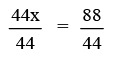or

x = 2

∴ The number = 31 * 2
= 62

Ques 5: Shobo’s mother’s present age is six times Shobo’s present age. Shobo’s age five years from now will be one-third of his mother’s present age. What are their present ages?
Ans: Let Shobo’s present age = x years

∴ Mother’s present age = 6x years
After 5 years:  Shobo’s age = (x + 5) years

Mother ’s age = (6x + 5) years

According to the condition, we have
1/3(Mother’s present age) = (Shobo’s age after 5 years)

i.e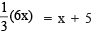or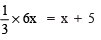or

2x = x + 5

or

2x – x = 5    (Transposing x to LHS)

or

x = 5

∴ Shobo’s present age = 5 years

Mother ’s present age = 6 * 5 = 30 years

Ques 6: There is a narrow rectangular plot, reserved for a school, in Mahuli village. The length and breadth of the plot are in the ratio 11 : 4. At the rate Rs 100 per metre it will cost the village panchayat Rs 75000 to fence the plot. What are the dimensions of the plot?
Ans: Let the
length = 11x metres
∴  Perimeter = 2(Length + Breadth)
= 2(11x + 4x)
= 2 * 15x
= 30x

∴  Cost of fencing = Rs 100 * 30x
= Rs 3000x

But the cost of fencing is Rs 75000.

∴  3000x = 75000.

or

x = 75000/3000 = 25

∴ Length = 11 * 25 = 275 metres

Breadth = 4 * 25 = 100 metres

Ques 7: Hasan buys two kinds of cloth materials for school uniforms, shirt material that costs him Rs 50 per metre and trouser material that costs him Rs 90 per metre. For every 2 metres of the trouser material he buys 3 metres of the shirt material. He sells the materials at 12% and 10% profit respectively. His total sale is Rs 36,600. How much trouser material did he buy?
Ans: Let the length of cloth for trousers = 2x metres

∴ The length of cloth for shirts = 3x metres

Cost of trouser’s cloth = 2x * Rs 90
= Rs 180x
Cost of shirt’s cloth = 3x * Rs 50
= Rs 150x

S.P. of trouser ’s cloth at 10% profit =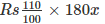= Rs 198x

S.P. of shirt’s cloth at 12% profit =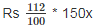= Rs 168x

∴ Total S.P. = Rs 198x + Rs 168x
= Rs 366x

But the total S.P. = Rs 36600

∴ 366x = 36600

or

x = 36600/366     [Dividing both sides by 366]
= 100

∴ 2x = 2 * 100 = 200

Thus, he bought cloth for trousers = 200 metres.

Ques 8: Half of a herd of deer are grazing in the field and three-fourths of the remaining are playing nearby. The rest 9 are drinking water from the pond. Find the number of deer in the herd.
Ans: Let the total number of deer = x

∴  Number of deer: Grazing in the field = x/2

Playing nearly =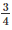x (Remaining no of deer)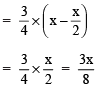Drinking water = 9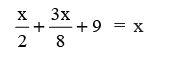Transposing 9 to RHS, and x to LHS, we have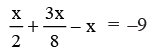or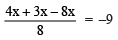[∵ LCM of 2 and 8 is 8]

or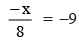Multiply by 8 on both sides, we have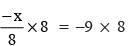or

–x = –72 ⇒ x = 72

Thus, the number of deer in herd = 72

Ques 9: A grandfather is ten times older than his granddaughter. He is also 54 years older than her. Find their present age.
Ans: Let the present age of grandfather = x years

∴ Present age of granddaughter =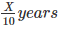According to the condition, we have
[Present age of granddaughter] + 54 = x

or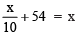Transposing 54 to RHS and x to LHS, we have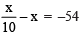or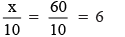or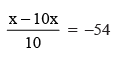or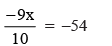or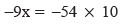[Multiplying both sides by 10]

or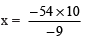[Dividing both sides by –9]

or

x = 6 * 10 = 60

and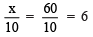∴ Present age of grandfather = 60 years
Present age of granddaughter = 6 years

Ques 10: Aman’s age is three times his son’s age. Ten years ago he was five times his son’s age. Find their present ages.
Ans: Let present age of Son = x years

∴  present age of Aman = 3x years
Ten years ago Son’s age = (x – 10) years
Aman’s age = (3x – 10) years

According to the condition, we have

[Son’s age (10 years ago)] * 5 = [Aman’s age (10 years ago)]

or

(x – 10) * 5 = (3x – 10)

or

5x – 50 = 3x – 10

Transposing (–50) to RHS and 3x to LHS, we have
5x – 3x = –10 + 50

or

2x = 40

or  x = 40/2 = 20     [Dividing both sides by 2]

and 3x = 3 * 20 = 60

∴  Son’s present age = 20 years

Aman’s present age = 60 years.

Offer running on EduRev: Apply code STAYHOME200 to get INR 200 off on our premium plan EduRev Infinity!

93 docs|16 tests

,

,

,

,

,

,

,

,

,

,

,

,

,

,

,

,

,

,

,

,

,

;